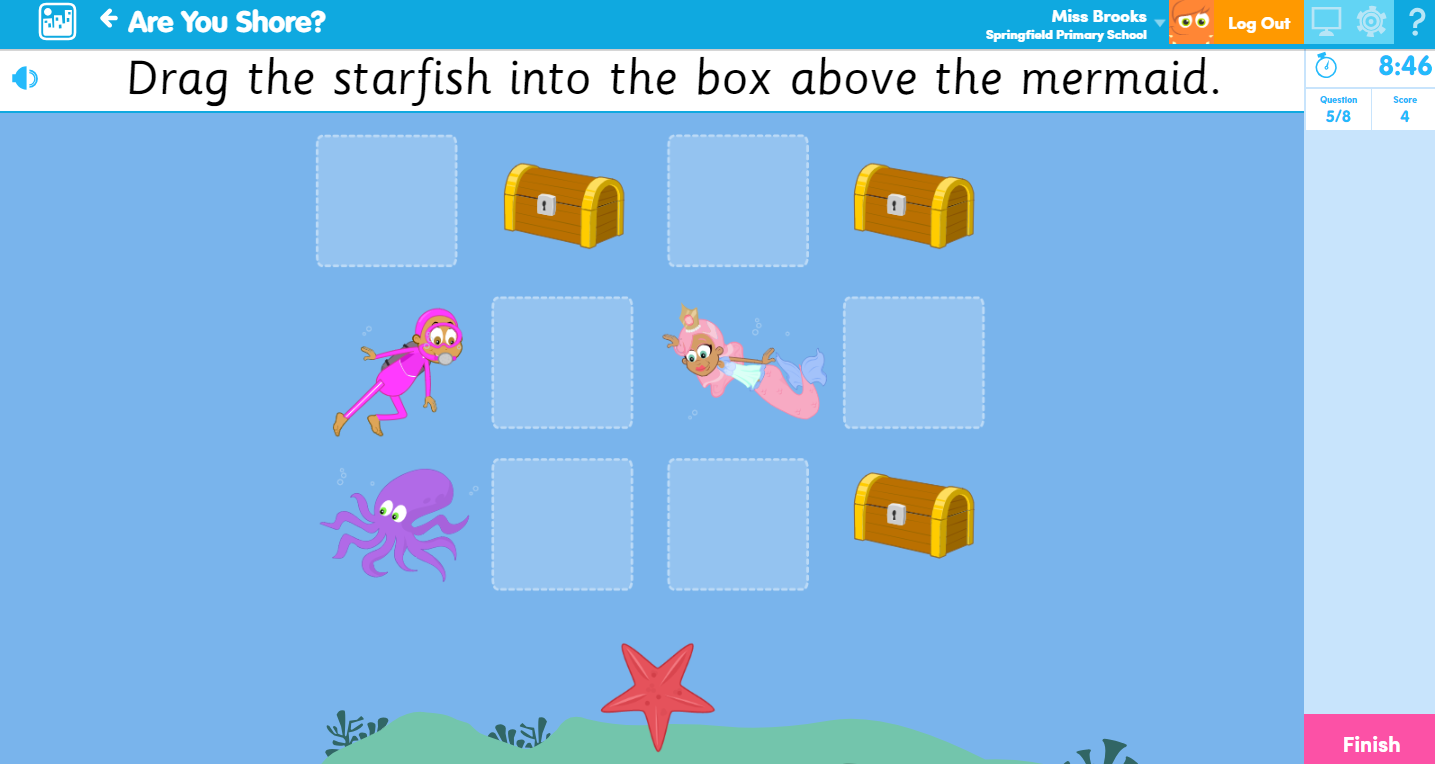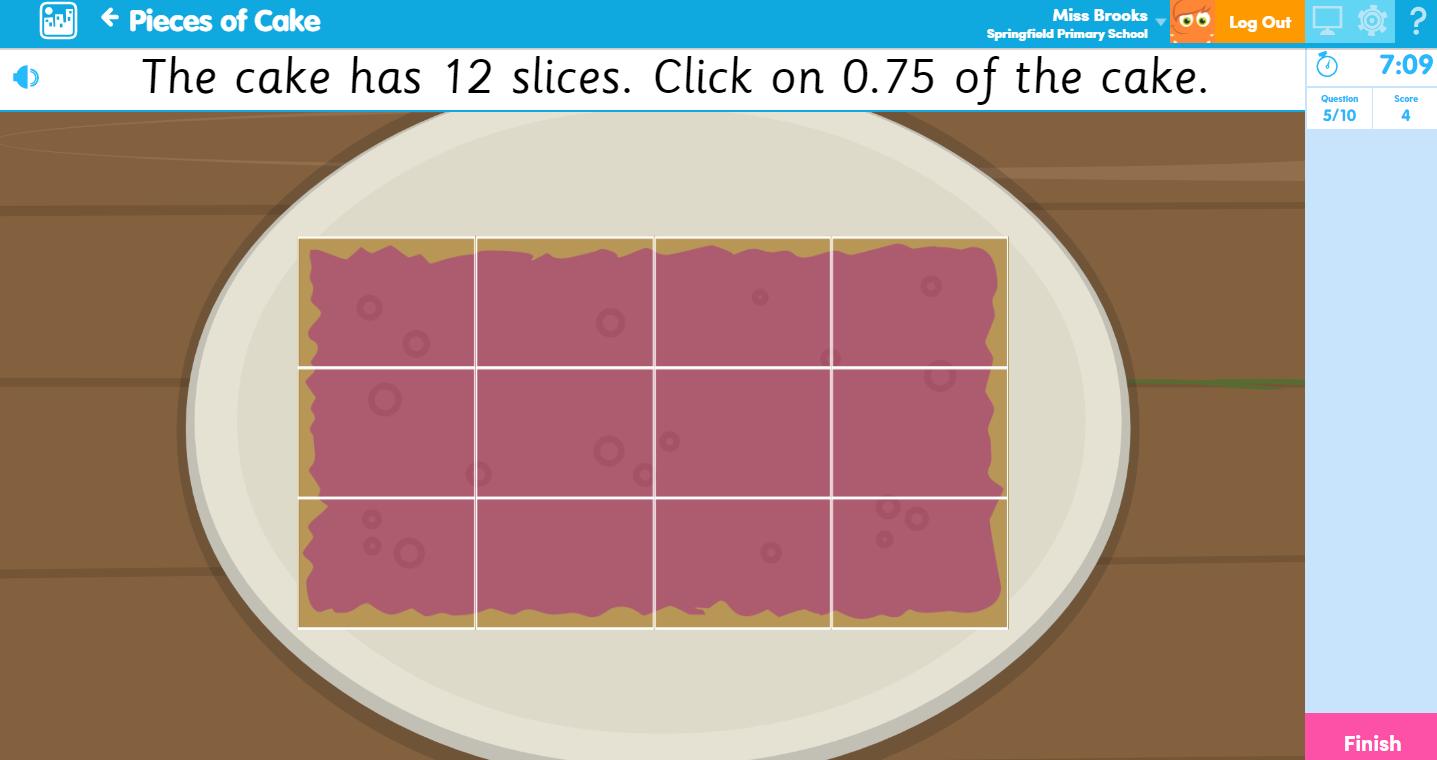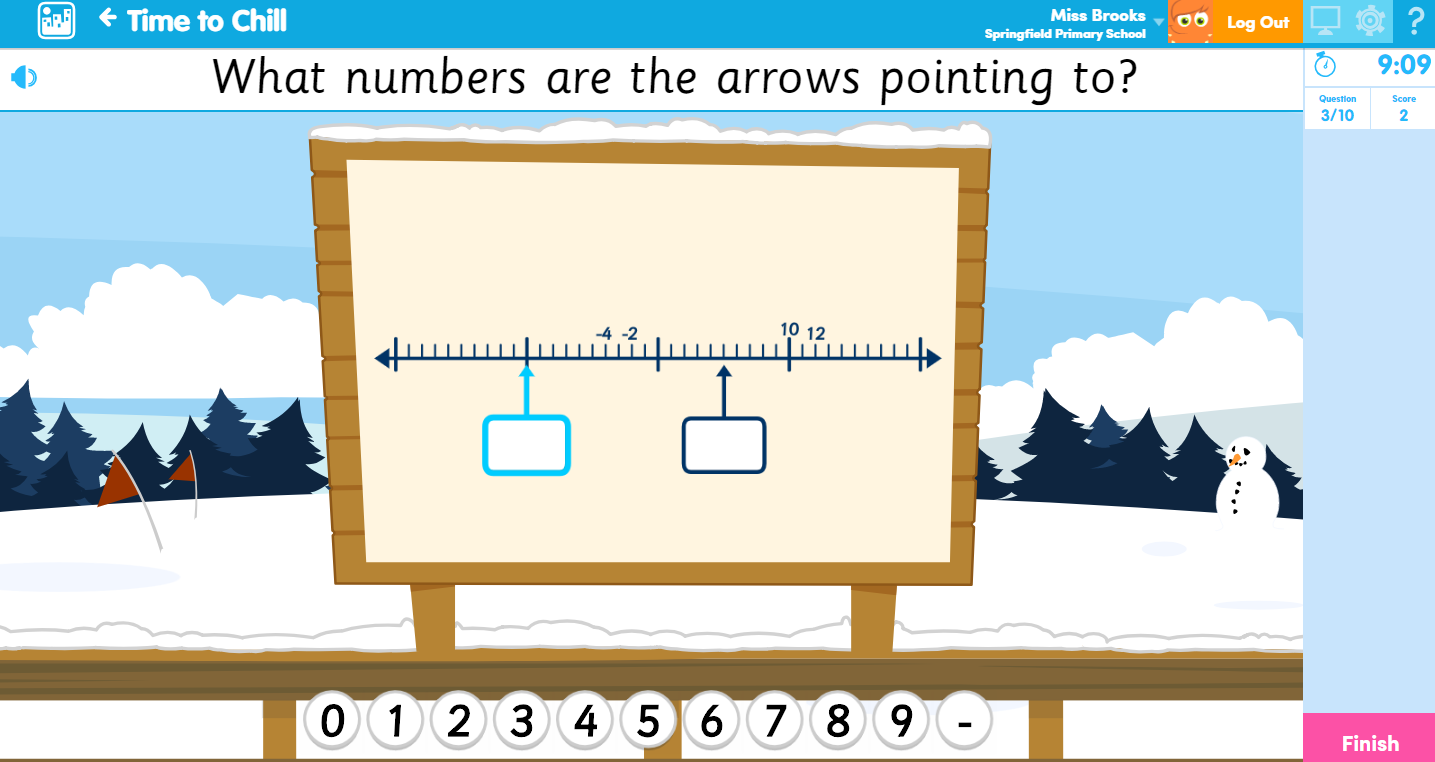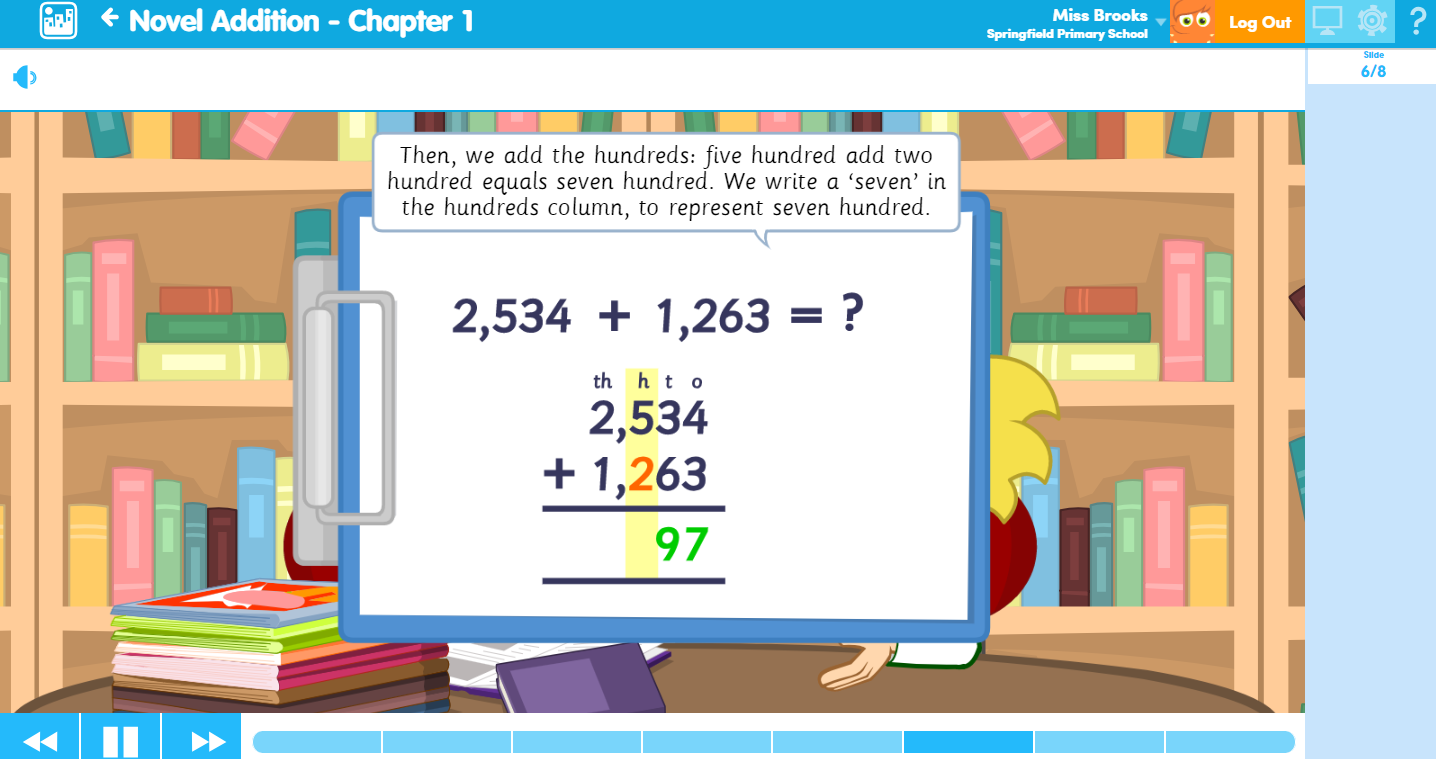# New Maths Content

Take a look below at some of the new resources we’ve picked out for you to try – you can see the rest in the New Content area when you log in.

Please note that the content listed below is arranged according to the National Curriculum in England.

Maths

Activities

Key Stage 1Are You Shore?: #32816 – This Activity focuses on the following positional language: left, right top, middle, bottom, on top of, above, below, under, between.

Plaice Value: #32782 – Identify and represent numbers from 0 to 100, using place value blocks.

Lower Key Stage 2

Carnival Graphs: #34914 – Interpret and present data in bar charts and time graphs to solve problems.

Change of Scene: #34667 – Converting between different units of measure including: time, length, volume and mass.

Fractions to Go!: #34638 – Use diagrams to recognise and show equivalent fractions with small denominators.

Hole Number Fishing: #34629 – Round decimals with one decimal place to the nearest whole number.

In Safe Hands: #34499 – Tell and write the time from an analogue, 12- hour and 24- hour clock.

On the Rocks: #34545 – Fill in the missing numbers in the number patterns. Answer the questions about the number patterns.

Pieces of Cake: #34542 – Students are taught to recognise the decimal equivalence of the fractions ¼, 1/2, and ¾.Pizza Box Fractions: #34532 – Students answer a selection of different fraction problems. Questions include ordering unit fractions and fractions with the same denominator, recognising equivalent fractions, finding a fraction of a discrete set of objects and adding and subtracting fractions.

Shapes in the Sand: #34548 – Identify lines of symmetry in 2-D shapes in different orientations.

Thirds of a Feather: #34929 – Help Sten to find non-unit fractions of different quantities.

This Scampi Happening: #32783 – Identify and represent numbers from 0 to 1,000, using place value blocks.

Time Bandit: #34926 – Convert time between analogue and digital 12 and 24-hour clocks.

Time to Chill: #34820 – Count back through zero to include negative numbers.

Upper Key Stage 2

Bargain Hunter: #34617 – Recall and use equivalences between simple fractions, decimals and percentages, including in different contexts.Folding Space: #34923 – Identify the different nets of 3-D shapes and their features.

Match Day: #34938 – Identify and describe the position of a reflected shape.

Out on a Limb: #34676 – Help Sten build a treehouse by calculating lengths and perimeters. Calculations involve the conversion of units between centimetres and metres, and include decimals with up to 3 decimal places.

Party Problems: #34886 – Calculate with measurements.

Positional Play: #34935 – Identify and describe the position of a shape following a translation.

Ratio Rations: #34620 – Solve shop themed problems, involving the relative size of 2 quantities, where missing values can be found using multiplication and division facts. Reference given to ratio and the use of a:b notation.

Secret Formula: #34889 – Use given formulae to find the perimeter and area of triangles, squares, rectangles, parallelograms and regular polygons and the volume of cubes and cuboids.

Shed Shapes: #34932 – Calculate the perimeter of composite shapes.

Sun, Sand and Shopping: #34911 – Use estimation to check if Sten and his friends have enough money to buy the items they want in the beach shop. Then use estimation strategies in different real life scenarios with the summer holiday theme.Learn Screens

Lower Key Stage 2

Novel Addition – Chapter 1: #34893 – An introduction into formal written methods of columnar addition. This Learn Screen does not look at how to carry numbers.

Novel Addition – Chapter 2: #34894 – An introduction into formal written methods of columnar addition. This Learn Screen looks at how to carry numbers.

Novel Subtraction – Chapter 1: #34895 – An introduction into formal written methods of columnar subtraction. This Learn Screen does not look at exchanging.

Novel Subtraction – Chapter 2: #34896 – An introduction into formal written methods of columnar subtraction. This Learn Screen looks at exchanging.

Upper Key Stage 2

Bargains Galore: #34892 – Use knowledge of fractions and decimals to help work out a percentage of a whole number.